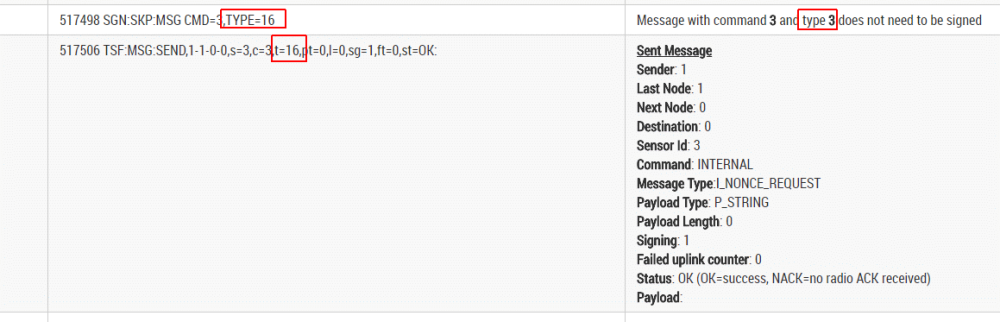# 💬 Log Parser

• This thread contains comments for the article "Log Parser" posted on MySensors.org.

• This post is deleted!

• I crash parser

``````0 MCO:BGN:INIT REPEATER,CP=RNORAA-,VER=2.1.1
4 TSM:INIT
4 TSF:WUR:MS=0
11 TSM:INIT:TSP OK
13 TSF:SID:OK,ID=15
15 TSM:FPAR
51 TSF:MSG:SEND,15-15-255-255,s=255,c=3,t=7,pt=0,l=0,sg=0,ft=0,st=OK:
2061 TSM:FPAR
2097 TSF:MSG:SEND,15-15-255-255,s=255,c=3,t=7,pt=0,l=0,sg=0,ft=0,st=OK:
2615 TSF:MSG:FPAR OK,ID=5,D=2
3057 TSF:MSG:FPAR OK,ID=0,D=1
4105 TSM:FPAR:OK
4106 TSM:ID
4107 TSM:ID:OK
4109 TSM:UPL
4146 !TSF:MSG:SEND,15-15-0-0,s=255,c=3,t=24,pt=1,l=1,sg=0,ft=0,st=NACK:1
6153 TSM:UPL
6190 !TSF:MSG:SEND,15-15-0-0,s=255,c=3,t=24,pt=1,l=1,sg=0,ft=1,st=NACK:1
8197 TSM:UPL
8233 !TSF:MSG:SEND,15-15-0-0,s=255,c=3,t=24,pt=1,l=1,sg=0,ft=2,st=NACK:1
10241 TSM:UPL
10244 TSF:MSG:SEND,15-15-0-0,s=255,c=3,t=24,pt=1,l=1,sg=0,ft=3,st=OK:1
10255 TSF:MSG:PONG RECV,HP=1
10258 TSM:UPL:OK
10267 TSF:MSG:SEND,15-15-0-0,s=255,c=4,t=0,pt=6,l=10,sg=0,ft=0,st=OK:FFFFFFFF40016FF10300
10311 !TSF:MSG:SEND,15-15-0-0,s=255,c=3,t=15,pt=6,l=2,sg=0,ft=0,st=NACK:0100
12322 TSF:MSG:SEND,15-15-0-0,s=255,c=0,t=18,pt=0,l=5,sg=0,ft=1,st=OK:2.1.1
12331 TSF:MSG:SEND,15-15-0-0,s=255,c=3,t=6,pt=1,l=1,sg=0,ft=0,st=OK:0
12578 TSF:MSG:SEND,15-15-0-0,s=255,c=3,t=11,pt=0,l=8,sg=0,ft=0,st=OK:Lighting
12597 TSF:MSG:SEND,15-15-0-0,s=255,c=3,t=12,pt=0,l=3,sg=0,ft=0,st=OK:1.2
12613 TSF:MSG:SEND,15-15-0-0,s=1,c=0,t=3,pt=0,l=11,sg=0,ft=0,st=OK:Light relay
12628 TSF:MSG:SEND,15-15-0-0,s=2,c=0,t=6,pt=0,l=15,sg=0,ft=0,st=OK:Key temperature
12641 TSF:MSG:SEND,15-15-0-0,s=3,c=0,t=30,pt=0,l=14,sg=0,ft=0,st=OK:Source current
``````

• Ok, fixed the problem. Thanks for reporting.

• Hi, I wonder why the following is parsed as a S_LIGHT presentation. Shouldn't be S_BINARY the type number 3? (https://www.mysensors.org/download/serial_api_20). Thanks!

``````0;1;0;0;3;
``````

• @user2684

Yes, it should be S_BINARAY. Thanks for reporting. Updated.

• I have just discovered "The Log Parser" !!!

WOW that's huge !

Thumbs up to those involved in that !!! Thanks a lot• The link "More details about the log messages." don't work. 404

• @raptorjr thanks for reporting. Could you describe where this link exists?
Edit: Found it. The link is fixed now. Thanks!

• @mfalkvidd I'm sorry. Thought it was obviuos since I posted in the comment section of the article where the link wasBut I will be more clear if I find a problem some other time.

Thanks for fixing the link.

• I think the log parser needs to be updated for new message types in MySensors v2.2.0 - they are currently being shown in the log parser as "Undefined":

I_SIGNAL_REPORT_REQUEST = 29,
I_SIGNAL_REPORT_REVERSE = 30,
I_SIGNAL_REPORT_RESPONSE = 31,

Is the source code for this parser somewhere on Github? If not, could we get it put on Github so it's easier to maintain?

• @Wes,

Here's a gist with the code for the log parser

• @hek I believe this should fix the issue:

https://gist.github.com/liwenyip/de6ff2a646ee417fcf2629eae64e6974/revisions#diff-a83c26f29ff664a062308cb4387554c0

AFAIK you can't do a pull request on Github Gists.

Hope that helps.

• Thanks, updated.

• Seems like log parser incorrectly prints command types for signature messages (see picture):• Just started MySensors 2.3 project. I have the same problem as Nca78 had 5 months again.
Only the two right columns are filled. Better than nothing but not perfect as intended.
And yes I tried running this code directly: https://gist.github.com/henrikekblad/c1d652cce16eaf2ef6340eacaefc97b0
Is anyone maintaining this code?

• Clearly the debug log format has changed to something that this tool does not support.
My log entries look like:
228642 TSF:MSG:SEND,1-1-0-0,s=2,c=1,t=16,pt=1,l=1,sg=0,ft=0,st=OK:0
The old log entries looked like this:

• The gist has now been updated to the lastest running on mysensors.org

• think it's time to add passive node support||| Unknown | ?TSF:MSG:SEND... | |

• No support for Gateway Transport messages?
GWT:TPS:TOPIC=mygateway1-out/19/255/3/0/21,MSG SENT